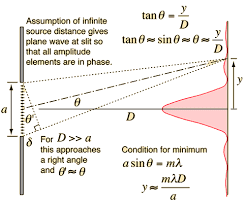# How's amplitude of a wave related to the slit width

## Homework Statement

How is the amplitude of a wave affected if the slit separation remains same but slit width is
1.)Increased?
2.)Decreased?
and why?

## Homework Equations

Intensity is proportional to the square of amplitude

## The Attempt at a Solution

I consider the amplitude as allowed "fatness" of a wave. The more slit width there is, the more wave can be "fat" and so it will have a greater amplitude.

## Answers and Replies

As you wrote intensity is proportional to the square of amplitude.
Do you know the relation between intensity of wave and the width of slits?
The intensity of light due to a slit (source of light) is directly proportional to width of the slit.
Therefore if the two slits ##S_1## & ##S_2## have widths ##W_1## & ##W_2## respectively and ##I_1## & ##I_2## are intensities of light waves having amplitude ##a_1## and ##a_2## from respective slits then,

##\frac{I_1}{1_2}## ∝ ##\frac{a_1^2}{a_2^2}## ∝ ##\frac{W_1}{W_2}##

Yes, I know that. I am asking why does the amplitude increases with increasing slit width

Yes, I know that. I am asking why does the amplitude increases with increasing slit width
In single slit diffraction calculations, the resultant amplitude is obtained by dividing the slit width into a large number of equal segments. For each segment, the amplitude is taken proportionally equal and the resultant amplitude is found by superposition of amplitudes of all the segments at the point of consideration.
So amplitude should be proportional to slit width.Can you see division of the slit width into a large number of equal segments?

Was my answer helpful?